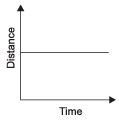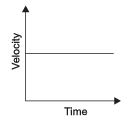Courses

# Motion, Science, Class 9 - Test

## 10 Questions MCQ Test Class 9 Science by VP Classes | Motion, Science, Class 9 - Test

Description
This mock test of Motion, Science, Class 9 - Test for Class 9 helps you for every Class 9 entrance exam. This contains 10 Multiple Choice Questions for Class 9 Motion, Science, Class 9 - Test (mcq) to study with solutions a complete question bank. The solved questions answers in this Motion, Science, Class 9 - Test quiz give you a good mix of easy questions and tough questions. Class 9 students definitely take this Motion, Science, Class 9 - Test exercise for a better result in the exam. You can find other Motion, Science, Class 9 - Test extra questions, long questions & short questions for Class 9 on EduRev as well by searching above.
QUESTION: 1

Solution:
QUESTION: 2

Solution:
QUESTION: 3

### The distance-time graph of an object is shown in the figure given below. The object isSolution:
QUESTION: 4

The area under the distance-time graph gives

Solution:
QUESTION: 5

The odometer of a car measures

Solution:
QUESTION: 6

The unit m/s2 is of

Solution:
QUESTION: 7

The velocity-time graph representsSolution:
QUESTION: 8

The area under velocity-time graph gives a physical quantity whose unit is

Solution:
QUESTION: 9

If a particle moves with a constant speed, the distance-time graph is

Solution:
QUESTION: 10

An object moving with uniform circular motion shows

Solution: